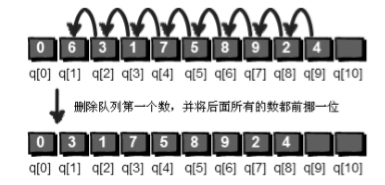### 珂朵莉

（快去看！出门左转樱x动漫！）$$\sum^k_{i=1}{c^2_i}$$

P1081

### 题目简述

A和B自左向右开车旅行（出发点可以变）

A、B的开车风格：

1.A会前往右边距他第二近的城市
2.B会前往右边最近的城市
3.A和B开过的总里程不超过$X$

1.给定一个$X$ 求出从哪个城市出发 $A$的路程/$B$的路程 最大

2.给定$X_i$和出发城市$S_i$ 求$A$和$B$分别开的距离数

## 题目描述

LCA就是LCA

### LCA的实现

1.让深的那个节点,$x$往树上爬，直到$deepth_x = deepth_y$；
2.让两个节点一起向上跳，如果碰在一起了，那么就找到了。

ST表的功能很简单：

### 什么是线段树

（1）求最值：给定$i$,$j<=n$，求${a_i, …, a_j}$区间内的最值。
（2）修改元素：给定$k$和$x$，把$a_k$改成$x$。

### 队列：### 链表